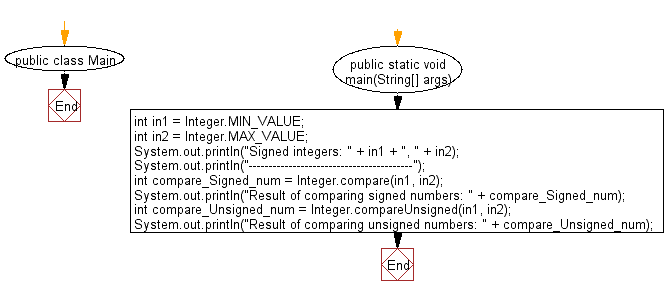﻿ Java exercises: Compare two given signed and unsigned numbers - w3resource# Java Data Type Exercises: Compare two given signed and unsigned numbers

## Java Data Type: Exercise-12 with Solution

Write a Java program to compare two given signed and unsigned numbers.

Sample Solution:

Java Code:

``````public class Main {
public static void main(String[] args) {
int in1 = Integer.MIN_VALUE;
int in2 = Integer.MAX_VALUE;
System.out.println("Signed integers: " + in1 + ", " + in2);
System.out.println("-----------------------------------------");
int compare_Signed_num = Integer.compare(in1, in2);
System.out.println("Result of comparing signed numbers: " + compare_Signed_num);
int compare_Unsigned_num = Integer.compareUnsigned(in1, in2);
System.out.println("Result of comparing unsigned numbers: " + compare_Unsigned_num);
}
}
```
```

Sample Output:

```Signed integers: -2147483648, 2147483647
-----------------------------------------
Result of comparing signed numbers: -1
Result of comparing unsigned numbers: 1
```

Flowchart:Java Code Editor:

Improve this sample solution and post your code through Disqus

What is the difficulty level of this exercise?

﻿

## Java: Tips of the Day

Checks if a string is upper case:

```public static boolean isUpperCase(String input) {
return Objects.equals(input, input.toUpperCase());
}
```

Ref: https://bit.ly/39Hpo84